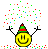# Inequalities in the real numbers

Gold Member
TL;DR Summary
It's so a basic question that I feel shame.
How do we get ##\epsilon(2p+\epsilon)<\epsilon(2p+1)<2-p^2## from ##0<\epsilon<1## and ##\epsilon<\dfrac{2-p^2}{2p+1}##?

Answer: As we have ##\epsilon<1##, we've got ##2p+\epsilon<2p+1##; therefore, ## \epsilon(2p+\epsilon)<\epsilon(2p+1) ##;
-as we have ##\epsilon<\dfrac{2-p^2}{2p+1}##, we also have ##\epsilon(2p+1)<2-p^2##. Is the red-coloured argue what I don't understand

Greetings, appreciated PF

Mentor
2022 Award
If ##\varepsilon < 1## then ##x+\varepsilon < x+1##. Set ##x=2p##. Then ##2p+\varepsilon <2p+1.## Now if ##a<b## then ##a\cdot \varepsilon < b\cdot \varepsilon ## because ##\varepsilon >0##. Now set ##a=2p+\varepsilon ## and ##b=2p+1## to get ##(2p+\varepsilon )\cdot \varepsilon < (2p+1)\cdot \varepsilon ##.

•mcastillo356
Gold Member
Fantastic, I never imagined it could be explained in so an easy way.
Thanks, fresh_42!

•fresh_42
Gold Member
Hi, PF, there is something more about this thread: I want to contextualize the question, and, by the way, ask another two questions. I beg you a pardon if this means to change the thread's place.

It's about Dedekind Cuts, concretely ## \sqrt 2 = \{r\in \mathbb Q\mid r<0\}\cup \{r\in \mathbb Q\mid r\geq 0,\ r^2<2\} ##

And the task is to prove ##\alpha=\sqrt 2## is a cut, without mentioning ##\sqrt 2##

1- As ##1^2<2<2^2##, it satisfies ##1\in\alpha## and ##2\in \mathbb Q\setminus\alpha##; so the first property of Dedekind Cuts is satisfied (for example, the proof that ##\alpha\neq \mathbb Q## is this: ##2\notin \alpha##, because it doesn't satisfy neither ##2<0## nor ## 2\geq 0 ## and ##2^2<2##).

2-If ##p\in\alpha## and ##q<p##, or ##q<0##, in which case ##p\in \alpha##, by definition, or ##0\leq q<p##; hence ##p^2<q^2<2##. Therefore, ##q\in \alpha##.
Why is that ##p^2<q^2<2##?

3- If ##p\in\alpha##, alternatively ##p\leq 0##, in which case ##p<1\in\alpha##, or ##p>0##, in which case ##p^2<2##. Let's see what must require a rational number ##\epsilon>0## so that ##p+\epsilon\in \alpha##:

##(p+\epsilon)^2=p^2+2p\epsilon+\epsilon^2<2##

This is equivalent to ##\epsilon(2p+\epsilon)<2-p^2##. If we take ##0<\epsilon<1## and ##\epsilon<\dfrac{2-p^2}{2p+1}## then
##2(p+\epsilon)<\epsilon(2p+1)<2-p^2##, so ##p<q=p+\epsilon\in \alpha##

Why this implication?

Greetings! Hope not to have treated too badly the english.

Homework Helper
Gold Member
2022 Award
3- If ##p\in\alpha##, alternatively ##p\leq 0##, in which case ##p<1\in\alpha##, or ##p>0##, in which case ##p^2<2##. Let's see what must require a rational number ##\epsilon>0## so that ##p+\epsilon\in \alpha##:

##(p+\epsilon)^2=p^2+2p\epsilon+\epsilon^2<2##

This is equivalent to ##\epsilon(2p+\epsilon)<2-p^2##. If we take ##0<\epsilon<1## and ##\epsilon<\dfrac{2-p^2}{2p+1}## then
##2(p+\epsilon)<\epsilon(2p+1)<2-p^2##, so ##p<q=p+\epsilon\in \alpha##

Why this implication?

Greetings! Hope not to have treated too badly the english.
I don't understand the bit in red at the end. You have:

##0<\epsilon<1## and ##\epsilon<\dfrac{2-p^2}{2p+1}##

Now you have to show that, with these bounds on ##\epsilon## we have ##(p + \epsilon)^2 < 2##. I.e. ##p + \epsilon \in \alpha##.

•mcastillo356
Gold Member
Hello, PeroK
The target consists in suppose ##p\in \alpha##, this is, we have ##p## that satisfies ##p<0## or ##p\geq 0## and ##p^2<2## (not mentioning ##\sqrt 2##), and I must find a ##q>p## that satisfies the same (whithout references to ##\sqrt 2##.
All I find in the proof is a dance of ##\epsilon##, ##p##, 0, 1, and 2.
Let's see, I think I understand:
##2(p+\epsilon)<(p+\epsilon)^2<2##: ##2(p+\epsilon)=q\in \alpha##

Homework Helper
Gold Member
2022 Award
Hello, PeroK
The target consists in suppose ##p\in \alpha##, this is, we have ##p## that satisfies ##p<0## or ##p\geq 0## and ##p^2<2## (not mentioning ##\sqrt 2##), and I must find a ##q>p## that satisfies the same (whithout references to ##\sqrt 2##.
All I find in the proof is a dance of ##\epsilon##, ##p##, 0, 1, and 2.
Let's see, I think I understand:
##2(p+\epsilon)<(p+\epsilon)^2<2##: ##2(p+\epsilon)=q\in \alpha##
I don't understand that at all.

You have to show that:

##0<\epsilon<1## and ##\epsilon<\dfrac{2-p^2}{2p+1} \ \ \Rightarrow \ \ (p + \epsilon)^2 < 2##

That should be quite simple.

•mcastillo356
Homework Helper
Gold Member
2022 Award
PS you could check it out for ##p = \frac 7 5##.

•mcastillo356
Gold Member
It works, but, how can I prove it algebraically?

Homework Helper
Gold Member
2022 Award
It works, but, how can I prove it algebraically?
Using algebra! Starting with ##(p + \epsilon)^2 = p^2 + 2p\epsilon + \epsilon^2##

•mcastillo356
Gold Member
Ok, processing...

Mentor
@mcastillo356, I revised your thread title slightly, changing "inequations" to "inequalities," which is what we call them in English. Inconsistent, but that's English for you.

•mcastillo356
Gold Member
You have to show that:

##0<\epsilon<1## and ##\epsilon<\dfrac{2-p^2}{2p+1} \ \ \Rightarrow \ \ (p + \epsilon)^2 < 2##

That should be quite simple.

Using algebra! Starting with ##(p + \epsilon)^2 = p^2 + 2p\epsilon + \epsilon^2##

I know only to prove this:
Dedekind Cuts, third property:
III If ##p\in\alpha## then ##p<r## for some ##r\in \alpha##
For example, the cut ##\sqrt 2 = \{r\in \mathbb Q\mid r\leq 0\}\cup \{r\in \mathbb Q\mid r> 0,\ r^2<2\}##. If ##p\in\alpha##, alternatively ##p\leq 0##, in which case ##p<1\in\alpha##, or ##p>0##, in which case ##p^2<2##. Let's see what must require a rational number ##\epsilon>0## so that ##p+\epsilon\in \alpha##:

##(p+\epsilon)^2=p^2+2p\epsilon+\epsilon^2<2##

This is equivalent to ##\epsilon(2p+\epsilon)<2-p^2##. If we take ##0<\epsilon<1## and ##\epsilon<\dfrac{2-p^2}{2p+1}## then we've found the proper ##\epsilon##.
Problem solved?

##2(p+\epsilon)<\epsilon(2p+1)<2-p^2## would be another way to express the two inequalities that bound ##\epsilon##

Homework Helper
Gold Member
2022 Award
If we take ##0<\epsilon<1## and ##\epsilon<\dfrac{2-p^2}{2p+1}## then we've found the proper ##\epsilon##.
Problem solved?
I would still like to see a direct calculation that starts with that ##\epsilon## and shows that ##(p+\epsilon)^2 < 2##.

•mcastillo356
Gold Member
I will try. I'll answer tomorrow

Gold Member
Hello, PeroK, PF
##0<\epsilon<1## and ##\epsilon<\dfrac{2-p^2}{2p+1}\Rightarrow{(p+\epsilon)^2<2}##
##0<\epsilon<\dfrac{2-p^2}{2p+1}##
##\Rightarrow{0<\epsilon(2p+1)<2-p^2}##
##\Rightarrow{p^2<2}##
Dedekind Cuts, third property
##\therefore{(p+\epsilon)^2<2}##?

Homework Helper
Gold Member
2022 Award
Hello, PeroK, PF
##0<\epsilon<1## and ##\epsilon<\dfrac{2-p^2}{2p+1}\Rightarrow{(p+\epsilon)^2<2}##
##0<\epsilon<\dfrac{2-p^2}{2p+1}##
##\Rightarrow{0<\epsilon(2p+1)<2-p^2}##
##\Rightarrow{p^2<2}##
Dedekind Cuts, third property
##\therefore{(p+\epsilon)^2<2}##?
We already knew that ##p^2 < 2##. It was ##(p+ \epsilon)^2 < 2## that must be shown.

I gave you the first step previously (which you ignored!): $$(p+\epsilon)^2 = p^2 + 2p\epsilon + \epsilon^2 = p^2 + \epsilon(2p + \epsilon)$$ The second step is: $$p^2 + \epsilon(2p + \epsilon) < p^2 + \epsilon(2p + 1) \ \ (\text{as} \ \ \epsilon <1)$$ Just two steps left for you now!

•mcastillo356
Gold Member
Hi, PeroK, PF

$$(p+\epsilon)^2=p^2+2p\epsilon+\epsilon^2=p^2+\epsilon(2p+\epsilon)$$

$$\epsilon<1\Rightarrow p^2+\epsilon(2p+\epsilon)<p^2+\epsilon(2p+1)$$

$$\epsilon<\dfrac{2-p^2}{2p+1}\Rightarrow \epsilon(2p+1)<2-p^2$$

$$p^2+\epsilon(2p+\epsilon)<p^2+\epsilon(2p+1)<2-p^2+p^2$$

$$\Rightarrow (p+\epsilon)^2<2\Rightarrow (p+\epsilon)\in \alpha$$

?•PeroK•